# Definition

A `connected scatterplot` displays the evolution of a numeric variable. Data points are represented by a dot and connected by straight line segments. It often shows a trend in data over intervals of time: a time series. Basically it is the same as a line plot in most of the cases, except that individual observation are highlighted.

The following example shows the evolution of the bitcoin price in April 2018. Data comes from the CoinMarketCap website.

``````# Libraries
library(tidyverse)
library(hrbrthemes)
library(plotly)
library(patchwork)
library(babynames)
library(viridis)

# Load dataset from github
data\$date <- as.Date(data\$date)

# Plot
data %>%
tail(10) %>%
ggplot( aes(x=date, y=value)) +
geom_line(color="#69b3a2") +
geom_point(color="#69b3a2", size=4) +
ggtitle("Evolution of Bitcoin price") +
ylab("bitcoin price (\$)") +
theme_ipsum()``````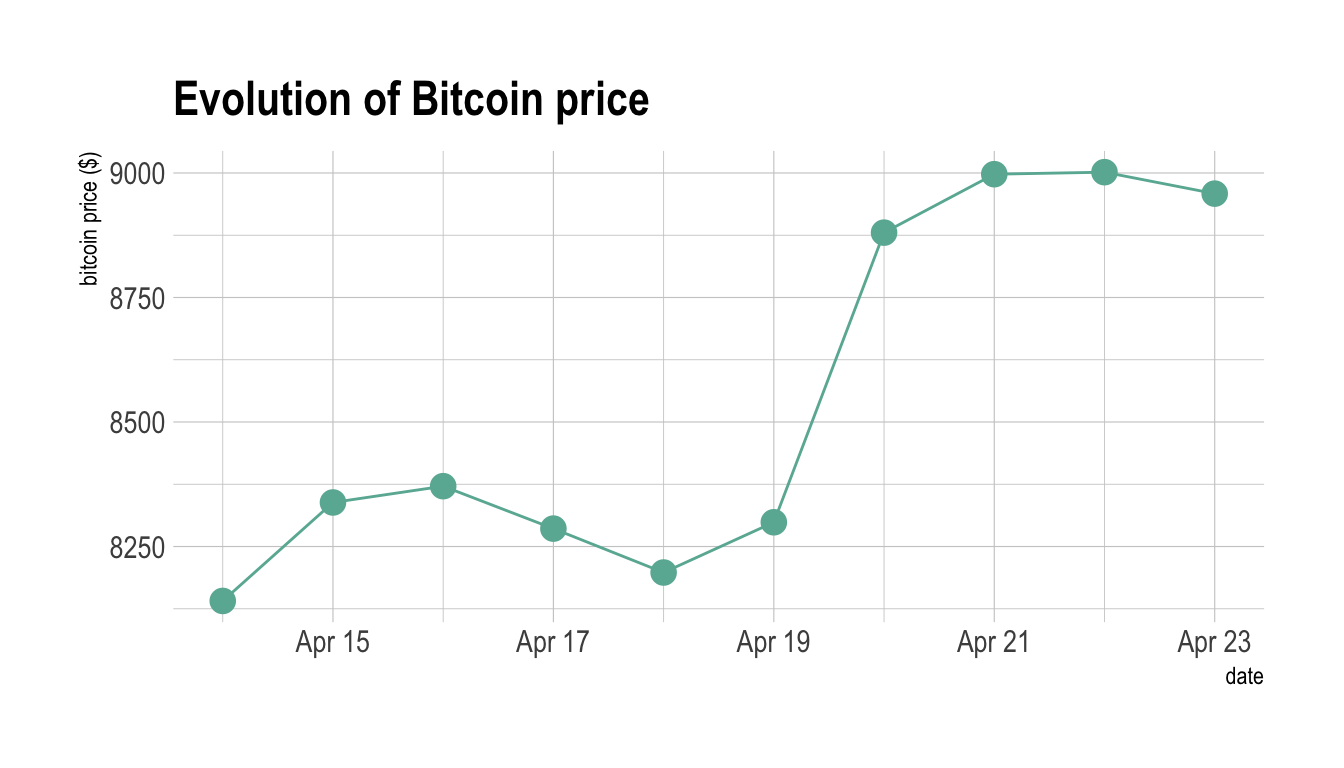# What for

Connected scatterplot makes sense in specific conditions where both the scatterplot and the line chart are not enough:

• When doing a line chart, it is sometimes difficult to visualize where the breaks in the curve are, and thus when the observation have been done.
``````# Plot
p1 <- data %>%
tail(60) %>%
ggplot( aes(x=date, y=value)) +
geom_line(color="#69b3a2") +
ggtitle("Line chart") +
ylab("bitcoin price (\$)") +
theme_ipsum()

p2 <- data %>%
tail(60) %>%
ggplot( aes(x=date, y=value)) +
geom_line(color="#69b3a2") +
geom_point(color="#69b3a2", size=2) +
ggtitle("Connected scatterplot") +
ylab("bitcoin price (\$)") +
theme_ipsum()

p1 + p2``````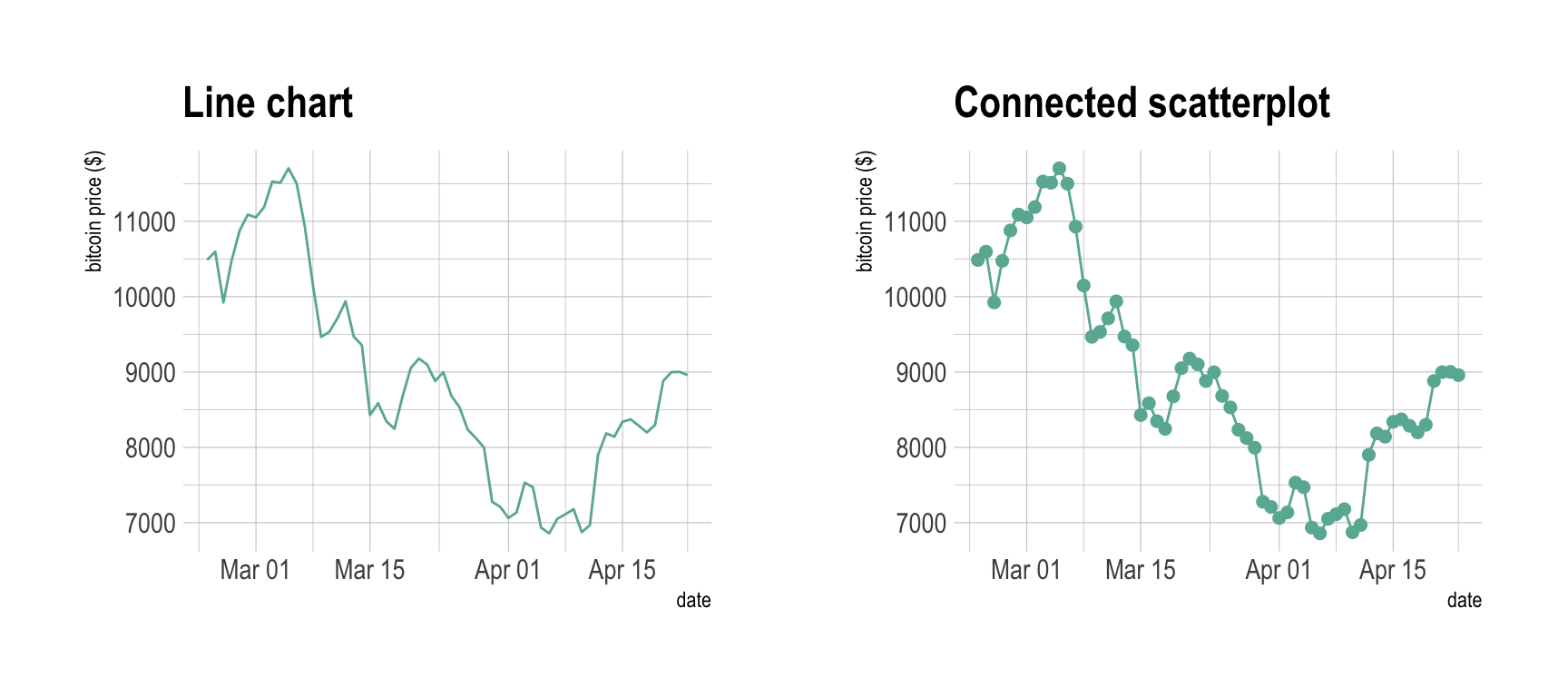• When your X axis is ordered you must connect the dots together to get a connected scatterplot. Indeed, the pattern is way hard to read if dots are not connected as illustrated in the graphic below. Note the this can even lead to misleading conclusion like explained here.
``````# Plot
data %>%
tail(60) %>%
ggplot( aes(x=date, y=value)) +
geom_point(color="#69b3a2", size=2) +
ggtitle("Line chart") +
ylab("bitcoin price (\$)") +
theme_ipsum()``````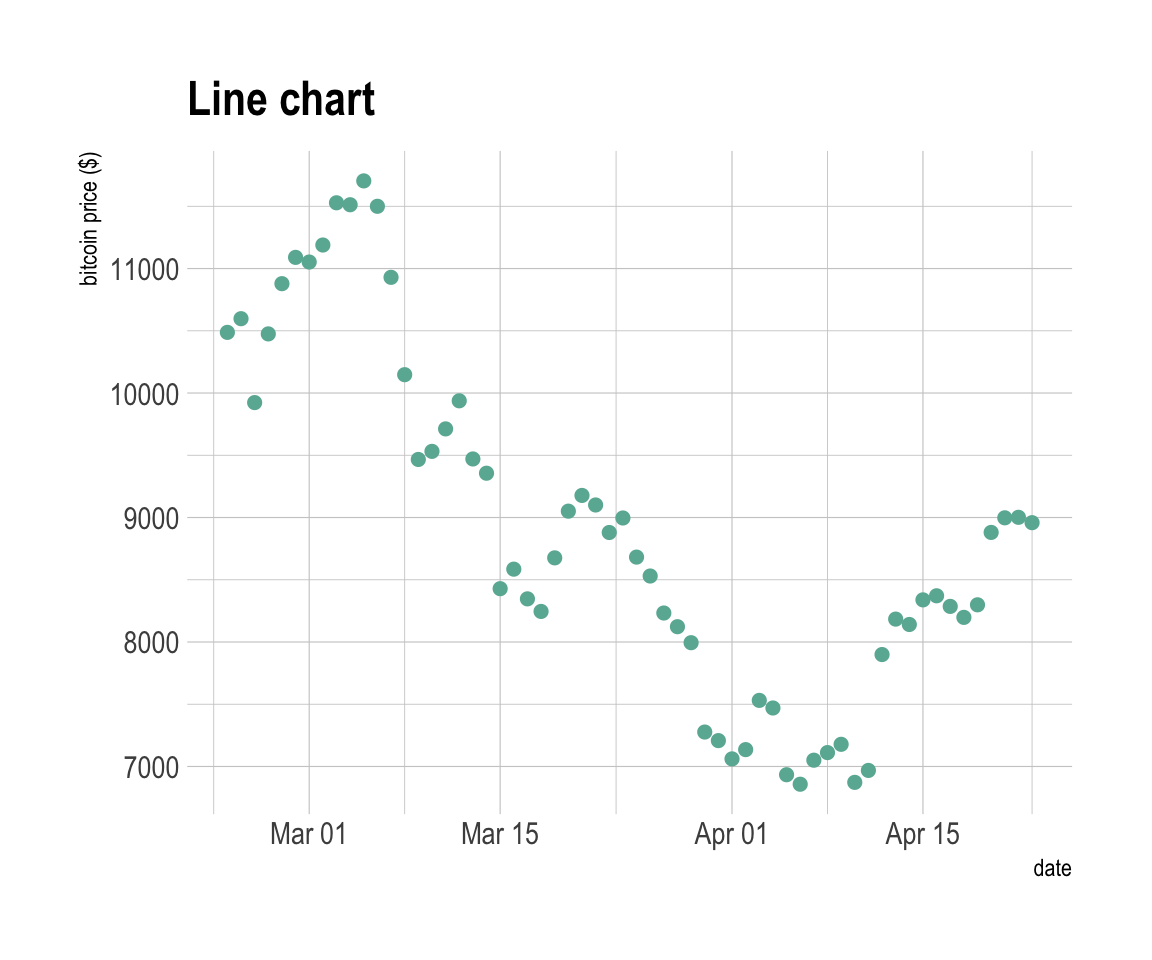# Variation

The connected scatterplot can also be a powerfull technique to tell a story about the evolution of 2 variables. Let’s consider a dataset composed of 3 columns:

• Year
• Number of baby born called Amanda this year
• Number of baby born called Ashley

We can first visualize the evolution of both names using a usual line plot with 2 lines:

``````library(babynames)

data <- babynames %>%
filter(name %in% c("Ashley", "Amanda")) %>%
filter(sex=="F")

#plot
data %>%
ggplot( aes(x=year, y=n, group=name, color=name)) +
geom_line() +
scale_color_viridis(discrete = TRUE, name="") +
theme(legend.position="none") +
ggtitle("Popularity of American names in the previous 30 years") +
theme_ipsum()``````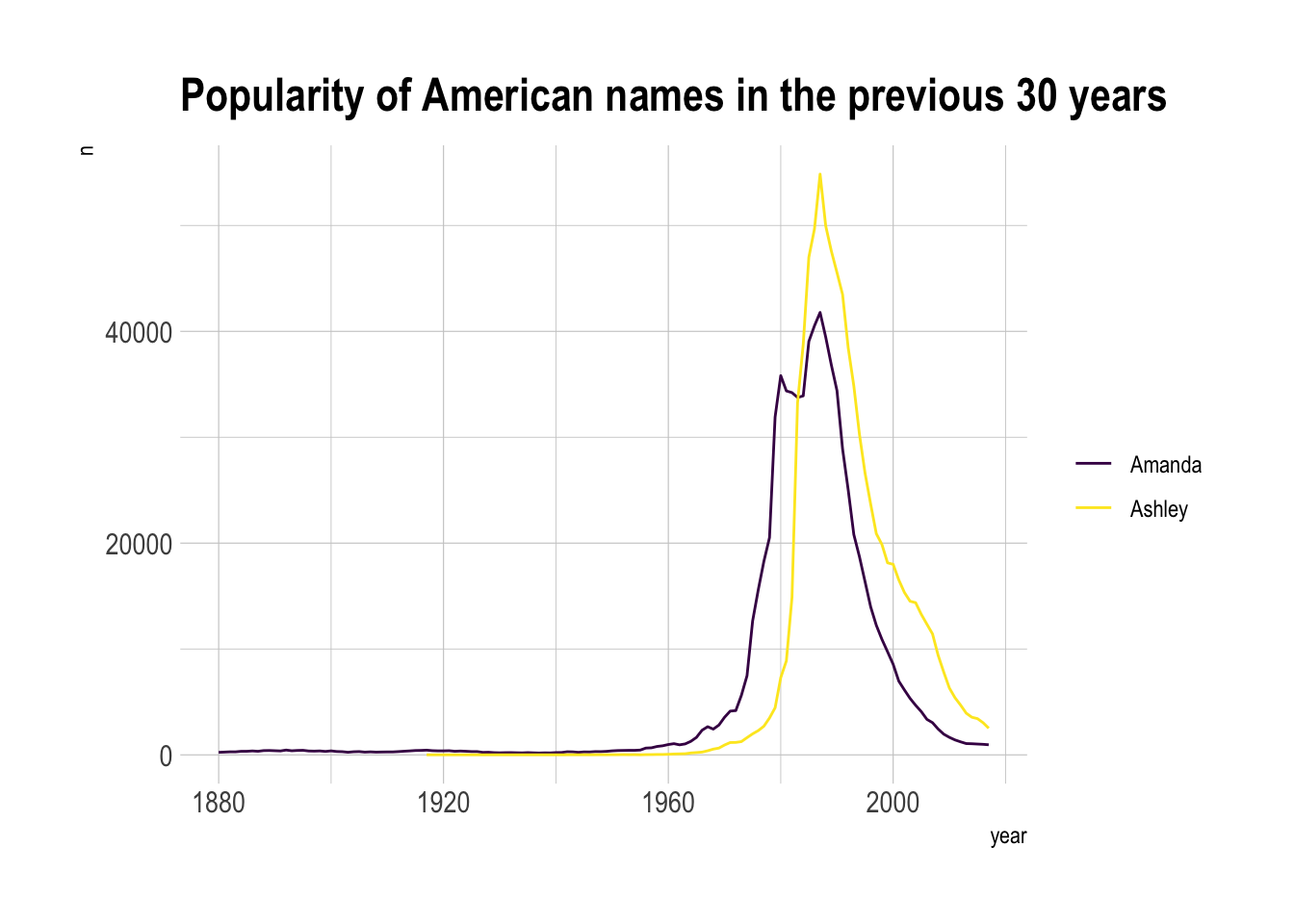This is an accurate way to visualize the information. However, it would be much harder to build it if both variables would not share the same unit. In this case, it would require a dual axis line chart that is known to be very misleading.

The connected scatterplot can be a good workaround in this situation:

``````library(grid) # needed for arrow function
library(ggrepel)

# data
tmp <- data %>%
filter(year>1970) %>%
select(year, name, n) %>%
spread(key = name, value=n, -1)

# data for date
tmp_date <- tmp %>% sample_frac(0.3)

tmp%>%
ggplot(aes(x=Amanda, y=Ashley, label=year)) +
geom_point(color="#69b3a2") +
geom_text_repel(data=tmp_date) +
geom_segment(color="#69b3a2",
aes(
xend=c(tail(Amanda, n=-1), NA),
yend=c(tail(Ashley, n=-1), NA)
),
arrow=arrow(length=unit(0.3,"cm"))
) +
theme_ipsum()``````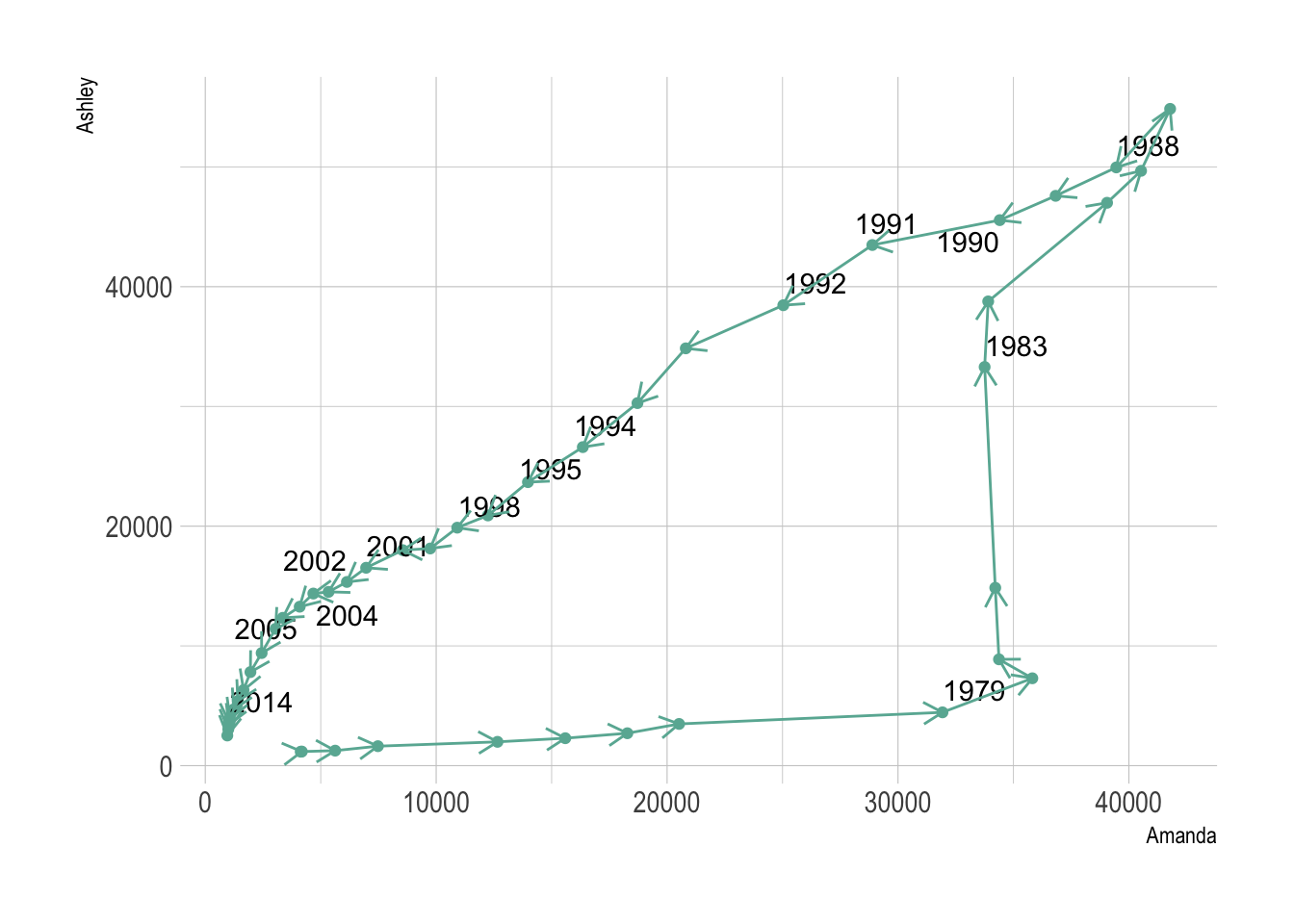Here the history of both names is obvious. They were not popular at all in 1972 at the beginning of the dataset. Between 1972 and 1980 Amanda got super popular but Ashley was still not common at all. Then a second period between 1980 is caracterized by the expansion of Ashley, Amanda remaining popular. Both names then decreased in popularity until 2014.

Warning: Readers usually expect time to flow from left-to-right. Here it flowed in both directions, what could mislead your audience. Here are a few advice:

• The direction of time should be strongly indicated. Arrows and date must be written on the chart.
• This graph is not adapted for all audience. At least, you need to educate the audience with progressive explanation to make it impactful.

Going further:

• The Connected Scatterplot for Presenting Paired Time Series by Haroz et al.
• A nice and famous example of story telling by the New York Times

# Common mistakes

The connected scatterplot is subject to the same caveats than the line chart:

• To cut or not to cut the Y axis? Wether or not the Y axis must start at 0 is a hot topic leading to intense debates. The graphic below presents the same data, starting at 0 (left) or not (right). Generally, line plot do not need to start at 0 since it allows to observe patterns more efficiently, but you probably want to learn more about it here.
``````data <- read.table("https://raw.githubusercontent.com/holtzy/data_to_viz/master/Example_dataset/3_TwoNumOrdered.csv", header=T)
data\$date <- as.Date(data\$date)

p1 <- data %>%
tail(10) %>%
ggplot( aes(x=date, y=value)) +
geom_line(color="#69b3a2") +
geom_point(color="#69b3a2", size=4) +
ggtitle("Not cuting") +
ylab("bitcoin price (\$)") +
theme_ipsum() +
ylim(0,10000)

p2 <- data %>%
tail(10) %>%
ggplot( aes(x=date, y=value)) +
geom_line(color="#69b3a2") +
geom_point(color="#69b3a2", size=4) +
ggtitle("Cuting") +
ylab("bitcoin price (\$)") +
theme_ipsum()

p1 + p2``````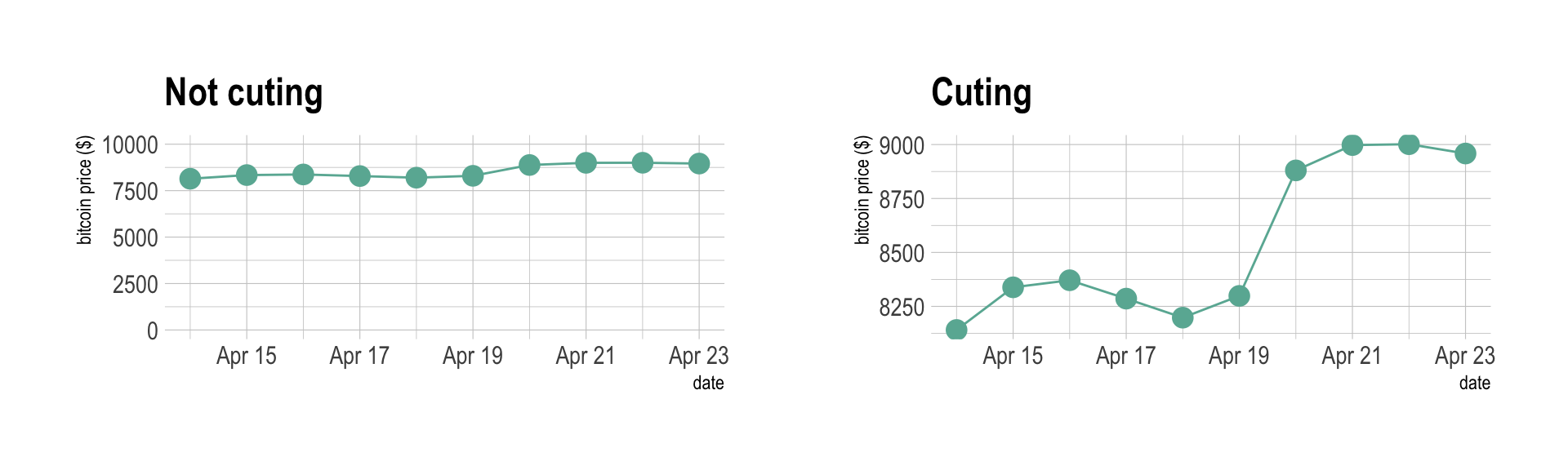• If you need to compare the evolution of 2 different variables, do not use dual axis. Indeed dual axis can show very different results depending on what range you apply to the axis. Read more about it.
• Mind the spaghetti chart: too many lines make the chart unreadable.
• Think about the aspect ratio of the graphic, extreme ratio make the chart unreadable.

# Build your own

The R and Python graph galleries are 2 websites providing hundreds of chart example, always providing the reproducible code. Click the button below to see how to build the chart you need with your favorite programing language.